Unsteady MHD Non-Newtonian Casson Fluid Flow due to a PorousRotating Disk with Uniform Electric Field | OMICS International
Fluid Mechanics: Open Access

# Unsteady MHD Non-Newtonian Casson Fluid Flow due to a PorousRotating Disk with Uniform Electric Field

Maleque A*

*Corresponding Author:
Kh. Abdul Maleque
Department of Mathematics
House - 23, Road - 17, Kemal Ataturk avenue
Tel: 88-02-8813233
E-mail: [email protected], [email protected]

Received Date: February 14, 2016; Accepted Date: February 25, 2016; Published Date: March 05, 2016

Citation: Maleque A (2016) Unsteady MHD Non-Newtonian Casson Fluid Flow due to a Porous Rotating Disk with Uniform Electric Field. Fluid Mech Open Acc 3:123. doi:10.4172/fmoa.1000123

Copyright: © 2016 Maleque A. This is an open-access article distributed under the terms of the Creative Commons Attribution License, which permits unrestricted use, distribution, and reproduction in any medium, provided the original author and source are credited.

Visit for more related articles at Fluid Mechanics: Open Access

#### Abstract

The unsteady MHD Non-Newtonain incompressible casson fluid flow due to a porous rotating disk with a uniform angular velocity in the presence of an axial uniform magnetic field and a uniform electric field is examined. The Hall current is not considered in this paper. The governing equations of the problem are then reduced to non-linear ordinary differential equations by introducing suitable similarity parameters. The similarity equations are derived in such a way that one can see the effects of the angular velocity explicitly unlike the usual similarity transformation of rotating disk problems. Numerical solutions to the reduced non-linear similarity equations are then obtained by adopting shooting method using the Nachtsheim-Swigert iteration technique.

#### Keywords

Rotaingdisk; Electric field; MHD flow; Non-Newtonian Casson fluid

#### Introduction

The equations of three dimensional viscous flows were first developed by Navier  and Poisson , on the basis of an argument which involved the consideration of intermolecular forces. Later the same equations were derived without the use of any such hypotheses by Venant  and Stokes . Von-Karman  has first discussed the rotating disk problem in 1921. Von-Karman’s original momentumintegral solution to the problem contained errors, which were pointed out by Cochran . Cochran reformulated the fifth-order system as a singular perturbation problem. He corrected Van-Karman’s solution and then calculated more accurate values by numerical integration of the equations. Benton  improved Cochran’s solution and extended the hydrodynamic problem to the flow starting impulsively from rest. In 1951, Howarth  discussed the equations of boundary layer flow in the vicinity of a separation point on general three dimensional case. He showed that the equations were reducible to pair of simultaneous ordinary third-order differential equations. Possible forms of similarity requirements irrespective of the body shape and its position in the direction of the motion were expressed in the tabular form by Hansan . He presented a table showing the nature of the variations in the main stream components for which the governing equations to a set of ordinary differential equations. Later for a mixed convection in three dimensional Cartesian system Maleque  and Zekerullah et al. [11,12] has showed that a restricted form of variation in temperature difference between the surfaceand the ambient fluid and the nature of free stream velocities are absolutely necessary to reduce the governing partial differential equations to a set of ordinary differential equations with transformed boundary conditions along with controlling parameters and then solved numerically. Similarity requirements are made by Zekerullah et al.  for convective boundary layer in orthogonal curvilinear surfaces and displayed in tabular form. A three dimensional boundary layer calculation is carried out by Allen et al.  for the flow over a semi-infinite circular cylinder. Al-Doss and Jerroch  dealt with the non Darcian mixed convection boundary layer flow about vertical cylinder. The effect of uniform blowing through a rotating porous disk on the flow induced by this disk was studied by Kuiken . Some interesting effects of the magnetic field on the steady flow due to the rotation of a disk of infinite or finite extent was examined by EL-Mistikawy et al. [17,18]. The steady magnetichydrodynamic boundary layer flow due to an infinite disk rotating with a uniform angular velocity in the presence of an axial magnetic field was investigated by Hassan et al. . Maleque et al. [20,21] investigated the effects of hall current and variable viscosity on an unsteady MHD laminar convective flow due to a rotating disk. Transient convective flow due to a rotating disk with magnetic field and heat absorption effects was studied by Maleque et al. . They also studied that the tangential component of the shear stress at the disk surface imparts of circumferential velocity to the adjacent fluid layer, which in turn, due to the centrifugal forces, also moves radially outwards . Steady MHD laminar convective fluid flow due to a porous rotating disk for compressible flow with variable properties was investigated by Maleque et al. [24,25]. Effects of combined temperature- and depth-dependent viscosity and hall current on an unsteady MHD laminar convective flow due to a rotating disk studied by Maleque [26,27]. Recently Maleque [28-31] investigated that Magnetohydrodynamic convective heat and mass transfer due to a rotating disk with thermal diffusion effect.

Above all works have been studied for Newtonian fluid flow. In many coating applications in the polymer processing industry especially in extraction of crude oil from petroleum products however fluids used to generate damage resistant surfacing are generally non-Newtonian. Several studied have been published concerning non-Newtonian flow by Acrivos et al. , Mitschka , Rogovskii et al.  and Sarma et al. . They presented a similarity solution for the power low fluid flow over a rotating disk. Rashaida et al.  considered both flow and species from a rotating disk to a Bingham platic. Recently Beg et al.  examined analytically the steady hydrodynamic flow of a power-low non-Newtonian fluid from a rotating disk to a saturated non-Darcian porous medium.

#### Governing Equations of the Flow Considered

Consider the unsteady MHD laminar boundary layer cassion fluid flow due to a rotating disk in an electrically conducting viscous incompressible fluid in the presence of an external magnetic field and uniform electric field. The equations governing for the Cassion fluid flow are

Equation of continuity: ∇.q = 0 (1)

Navier-Stokes Equation: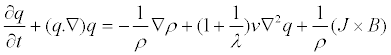(2)

The generalized Ohm’s law: J = σ [E + q × B − β (J × B)] (3)

The external uniform magnetic field is applied perpendicular to the plane of the disk and has a constant magnetic flux density B = (0, 0, B0) which is assumed unaltered by taking magnetic Reynolds number Rem << 1. E = (E0, E0, E0) is a uniform electric field which results from charge separation. The disk is assumed to be insulating and rotating in the z = 0 plane about the z = 0 axis with a uniform angular velocity Ω. The fluid is assumed to be incompressible and has density ρ, kinematic viscosity ν, electric conductivity σ and pressure is p and λ is the casson parameter, Due to the symmetric nature of the flow about the z = 0 plane, it is sufficient to consider the problem in the upper half space only. The physical configuration of the problem considered here is shown in the Figure 1. Assuming (u, v, w) as the components of the velocity vector q along the cylindrical polar coordinates (r, ?, z) respectively, the equation of continuity and Navier-Stokes equations take the form: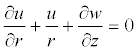(4)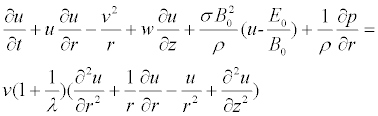(5)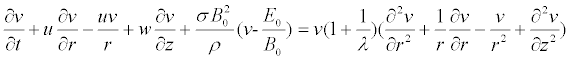(6)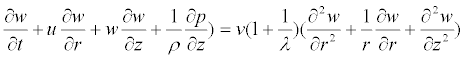(7)

The boundary conditions for the problem are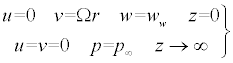(8)

#### Mathematical Formulations

To solve the governing equations (4)-(7), they are converted to some suitable form by introducing the following dimensionless quantities :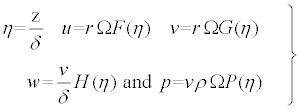(9)

where δ is a scale factor and is a function of time as δ = δ (t).

The above similarity transformations differ from the usual similarity transformation in the sense that the solutions would explicitly show the effects of rotation which are absent in the solutions obtained by usual transformations.

Then introducing the dimensionless quantities from equation (9) in equations (4)-(7) respectively, we obtain the following ordinary nonlinear differential equations .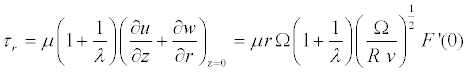(10)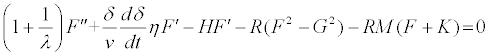(11)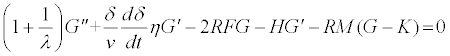(12)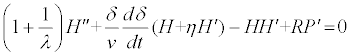(13)

Where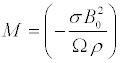, the magnetic interaction parameter,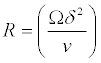the Reynolds number and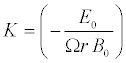the loading coefficient. K > 1 corresponds to the acceleration regime (of the pump), but K < 1, to the regime of breaking of flow. The interval of K values from 0 to +1 comprises the regime of the MHD generator .

The equations (10)-(13) are similar except for the term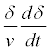where time t appears explicitly.

Thus the similarity condition requires thatmust be a constant quantity.

Hence following Maleque  one can try a class of solutions of the equations (10)-(12) by assuming that: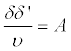(Constant) (14)

Integrating equation (13), we obtain: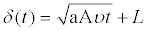(15)

where the constant of integration L is determined through the condition that δ = L when t = 0. Here A = 0 implies that δ = L represents the length scale for steady flow and A ≠ 0 that is, δ represents the length scale for unsteady flow For a class of solution Maleque  has considered A = 2. Since δ is a scaling factor as well as a similarity parameter, any other values of A in equation (14) would not change the nature of the solution except that the scale would be different. Finally, introducing equation (14) in equations (11)-(13) respectively, we have the following dimensionless ordinary non-linear differential equations

H’+ 2R F = 0 (16)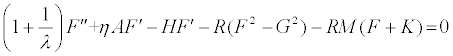(17)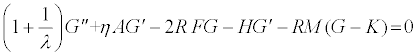(18)(19)

The boundary conditions (8) now transform to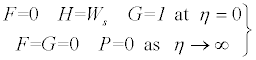(20)

Where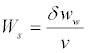and is obtained from equation (9). Here Ws represents a uniform suction (Ws < 0) or injection (Ws > 0) at the surface [24,25]. In all the above equations primes denote the differentiation with respect to η. Equations (16)-(18) are solved numerically under the boundary conditions (20) using Swigert  iteration technique.

Solution

Numericalsolutions to the transformed set of coupled, nonlinear, differential equations (17) and (18) were obtained, utilizing a modification of the program suggested by Nachtsheim and Swigert. Within the context of the initial value method and the Nachtsheim- Swigert iteration technique the outer boundary conditions may be functionally represented by the first order Taylor’s series as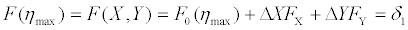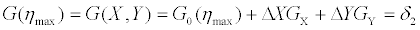with the asymptotic convergence criteria given by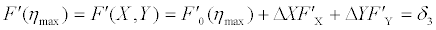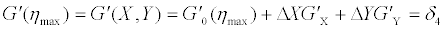where, X = F’(0), Y = G’(0), and X, Y subscripts indicate partial differentiation. e.g.,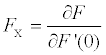. The subscript 0 indicates the value of the function at ηmax to be determined from the trial integration.

Solution of these equations in a least square sense requires determining the minimum value of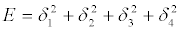with respect to X and Y. To solve ΔX and ΔY we require to differentiate E with respect to X and Y respectively. Thus adopting this numerical technique, acomputer program was set up for the solutions of the basic non-linear differential equations of our problem where the integration technique was adopted as a six ordered Range-Kutta method of integration. Various groups of the parameters γ, Ws, M and m were considered in different phases. In all the computations the step size Δη = 0.01 was selected that satisfied a convergence criterion of 10-6 in almost all of different phases mentioned above. Stating η = η + Δη, the value of η was found to each iteration loop. (η)max, to each group of the parameters, has been obtained when value of unknown boundary conditions at η = 0 not change to successful loop with error less than 10−6. However, different step sizes such as Δη = 0.01, Δη = 0.005 and Δη = 0.001 were also tried and the obtained solutions have been found to be independent of the step sizes as observed.

Equations (16)-(18) were then solved numerically by Nachtsheim- Swigert  iteration technique with the sixth order Range-Kutta integration scheme. The calculations were carried out for various values of casson parameter λ, suction/injection parameter Ws, Reynolds number R, Magnetic interaction parameter M and loading parameter K. From these solutions we may obtain derivatives of F and G functions at the disk surface (η = 0) which are required for the calculation of the tangential shear stress τt and the radial shear stress τr. To find the tangential shear stress τt and the radial shear stress τr, we apply the Newtonian formulae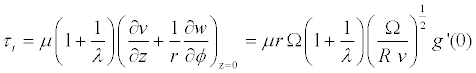andThe results of the numerical computations for the velocity profiles and the corresponding shearing stresses are presented graphically and in tabular form respectively.

#### Results and Discussions

The results of the numerical calculations are presented in the form of the radial velocity, tangential velocity and axial velocity profiles, which depict the effects of various parameters λ, K, Ws and M entering into the fluid flow due to the rotating disk. It is therefore, pertinent to enquire the effects of the variation of each of the parameter when the others are kept constant. Casson term may be neglected in the case λ → ∞ means the flow becomes Newtonian fluid on the other hand λ → 0 represents the highly non-Newtonian cassion fluid. In order to highlight the validity of the numerical computations adopted in the present investigation, some of our results for constant property case have been compared with those of Coachran  and Benton  in Table 1. The comparisons show excellent agreements, hence an encouragement for the use of the present numerical computations.

η F G H F G
0 0 1 0 0.5102646 0.6158157
0.1 0.0462779 0.9386031 0.0047921 0.4161761 0.6109809
0.5 0.1543452 0.7084644 0.0925489 0.1473909 0.5316866
1.0 0.1800178 0.4700941 0.2662011 -0.0161848 0.3913939
1.5 0.1562404 0.3134656 0.4350812 -0.0704791 0.2684607
2.0 0.1180752 0.2040024 0.5721281 -0.075267 0.1772014
3.0 0.0570782 0.080498 0.7457157 -0.0474911 0.0756797
4.0 0.0256194 0.034986 0.825765 -0.0230379 0.0316134
Results of Cochran 
0 0 1 0 0.51 0.616
0.1 0.046 0.939 0.005 0.416 0.611
0.5 0.154 0.708 0.092 0.147 0.532
1.0 0.18 0.468 0.266 -0.016 0.391
1.5 0.156 0.313 0.435 -0.07 0.268
2.0 0.118 0.203 0.572 -0.074 0.177
3.0 0.058 0.083 0.746 -0.046 0.075
4.0 0.026 0.035 0.826 -0.022 0.031
Results of Benton 
0 0 1 0 0.5102 0.6159
0.1 0.0462 0.9386 0.0048 0.4163 0.6112
0.5 0.1536 0.7076 0.0919 0.1467 0.5321
1.0 0.1802 0.4766 0.2655 -0.0157 0.3911
1.5 0.1559 0.3132 0.4357 -0.0693 0.2677
2.0 0.1189 0.2033 0.5732 -0.0739 0.1771
3.0 0.0581 0.0845 0.7452 -0.0455 0.0745
4.0 0.0257 0.0349 0.8251 -0.0216 0.0309

Table 1: Comparison of present result with Cochran  and Benton  and our present result.

Effect of casson parameter λ

Casson term is neglected for λ → ∞( = 1000) that means the flow becomes Newtonian fluid flow and λ → 0( = 0.1) represents the casson term is highly effected as well as the flow becomes highly non- Newtonian fluid flow. The effect of casson parameter λ on the radial, tangential and axial velocity profiles shown in Figures 2-4 respectively. It has been observed from Figure 2 that the velocity profiles decrease with the decreasing values of casson parameter λ. Also it has been investigated that for Newtonian fluid flow λ → ∞( = 1000) the boundary layer is closed to the disk and the boundary layer separations are found for non-Newtonian fluid. We have made the difference between the radial velocity profiles for λ → ∞( = 1000) and λ = 1.0 that the velocity profile decreases in the boundary layer 0 <η < 0.8 but decrease for η > 0.8 with the decreasing values of casson parameter λ and so on for λ = 0.2 and λ = 0.1. For decreasing values of λ lead to increase the boundary layer thickness. That is the radian frictional force decreases for strong casson fluid flow also agreed with Table 1. Figure 3 shows the effect of casson fluid parameter λ on tangential velocity profiles. The increase of λ leads to increase the velocity profiles. The velocity profile shows its usual trend of gradual decay for casson fluid flow. It is also investigated from this figure that for strong casson fluid the tangential boundary layer thickness is closed to the wall means high tangential frictional forces are appeared also shown in Table 2. The axial velocity increases with the increasing vales of λ shown in Figure 4.

R F′(0) G′(0) M (a) For M = 0.5 and λ=0.1 0.0 0.0 -1.15363 2.0 0.57187 -1.45922 4.0 0.9413 -1.82698 6.0 1.21441 -2.1493 8.0 1.43853 -2.43329 10.0 1.63254 -2.68902 (b) For R = 1.0 and λ= 0.1 0.0 0.24233 -1.16238 0.5 0.23635 -1.33993 1.0 0.23242 -1.50118 2.0 0.22842 -1.78734 3.0 0.22799 -2.03839 4.0 0.22766 -2.26385 (c) For M = 0.5 and R = 1 0.0001 0.0011069 -0.029523 0.001 0.0052883 -0.0508785 0.01 0.023714 -0.1189105 0.1 0.0797787 -0.3365182 0.5 0.1558607 -0.5981541 1 0.193284 -0.7070581 10 0.2805427 -0.9211743 ∞ 0.2805427 -0.9211743

Table 2: The radial and the tangential shearing stress τr and τt for the different values of R, M and λ.

Effect of magnetic parameter M

The parameter M do not enter directly into the equation (16), but it influences come through the solution of the equations (17) and (18). Figures 5-7 present the effect of the magnetic parameter M on the radial, the tangential and the axial velocity profiles for rotating parameter R = 1.0. The parameter M has marked effect on the velocity profiles. It is observed that F, G and H velocity profiles decrease by the increasing values of M. Increasing the magnetic interaction parameter has the effect of damping the fluidvelocity profiles. This is because the application of a transverse magnetic field normal to the flow direction will result in a resistive force (Lorentz force) similar to the drag force which tends to resist the fluid flow and thus reducing its velocity. Both tangential and radial shearing stresses decrease for increasing values of magnetic interaction parameter M shown in Table 2.

The effect of loading coefficient K on the radial, the tangential and the axial velocity profiles shown in Figures 8-10 respectively. K >1 corresponds to the acceleration regime (of the pump), but K < 1, to the regime of breaking of flow. The interval of K values from 0 to +1 comprises the regime of the MHD generator . The interval of K values from -1 to +1 are considered in our present paper. The radial velocity profile decreases for increasing values of loading coefficient K shown in Figure 7. For considering K = -1, Electric field is proportional to magnetic field (E0 = Ωr B0). Similar effects are found on radial velocity profiles for electric field and magnetic field. The tangential and axial velocity profiles increase with the increasing values of K shown in Figures 8 and 9 respectively.

Effect of suction/injection parameter Ws

The effects of suction and injection (Ws) for K = 0.05, M = 0.05 and λ = 0.5 on the radial, the tangential and the axial velocity profiles are shown in Figures 10-12. For suction Ws = -1, the axial velocity is nearly constant; the radial velocity is very small while tangential velocity decays rapidly away from the surface. The fact that suction stabilizes the boundary layer is also apparent from these figures. As for the injection (Ws > 0), from Figures 11-13 it is observed that the boundary layer is increasingly blown away from the disk to form an interlayer between the injection and the outer flow regions. From Figure 12 it is found that we observe that higher injection velocities have the tendency to destabilize the laminar flow. In Figure 11, it is observed that for high values of injection parameter (Ws = 4), the radial velocity near the disk (for small values of η ) is lower than that for smaller values of Ws. This is due to the fact that, with increasing values of Ws, the injected flow can sustain axial motion to greater distances from the wall. Then, near the wall, the radial flow which is fed by the axial flow is expected to decrease as the injected parameter increases. Opposite effects are found for strong suction Ws < -1.

A = 0 and A ≠ 0 represent the steady flow and unsteady flow respectively. The comparison of steady and unsteady flow on radial, tangential and axial velocities shown in Figure 14. The boundary layer thickness is close to the wall for unsteady flow.

The effects of various parameters (R and M) on the tangential and radial shearing stresses τt and τr are shown in Table 2. From Table 2, it is observed that the tangential shearing stress decreases and the radial shearing stress increases owing to the increase of rotating parameter R and magnetic interaction parameter M (Figure 15).

#### Conclusions

In this paper, the effects of casson parameter and electric field along with the effects of suction/injection on an unsteady MHD non Newtonian convective flow induced by an infinite rotating porous disk were studied. The following conclusions can be drawn as a result of the computations:

a. Casson parameter (λ) has marked effects on the radial, tangential and axial velocity profiles. Close to the surface of the disk these velocities slow down as λ increases but shortly after they increase with the increase of λ.

b. Due to the existence of the centrifugal force, the radial velocity reaches a maximum value close to the surface of the disk.

c. Close to the boundary positive values of λ is found to give rise to the familiar inflection point profile leading to the destabilization of the laminar flow. Strong injection also leads to the similar destabilization effect.

d. The effect of Lorentz force or the usual resistive effect of the magnetic field on the velocity profiles is apparent.

Increasing values of electric field (K) lead to decrease the boundary layer thickness.

#### References

Select your language of interest to view the total content in your interested language

### Article Usage

• Total views: 11918
• [From(publication date):
July-2016 - Dec 14, 2019]
• Breakdown by view type
• HTML page views : 11480Can't read the image? click here to refresh